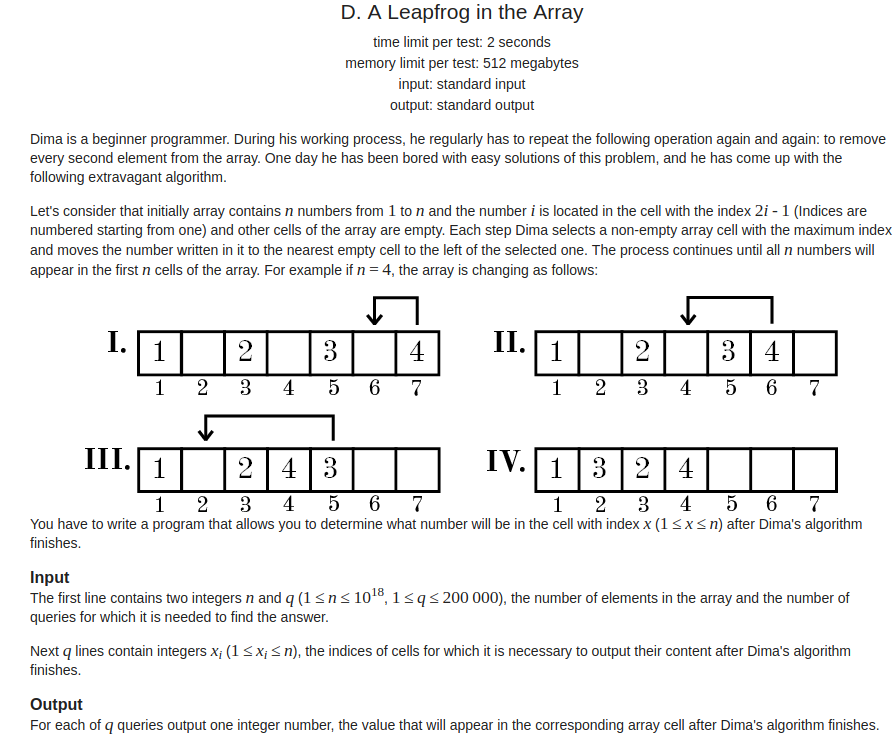Codeforces Round #469 (Div. 2) D. A Leapfrog in the Array (思维）Code

#include<cstdio>
#include<cstring>
#include<algorithm>
#include<iostream>
#include<cmath>
#include<vector>
#include<set>
#include<queue>
#include<limits.h>
#include<string.h>
using namespace std;
typedef long long ll;

#define inf int(0x3f3f3f3f)
#define mod int(1e9+7)
#define pi acos(-1.0)
#define lson  root << 1
#define rson  root << 1 | 1

ll n,q;

ll a;

int main()
{
ios::sync_with_stdio(false);
cin.tie(0);
cin>>n>>q;
for(int i=0;i<q;i++)
{
ll x;
cin>>x;
while(1)
{
if(x%2==1)
break;
x=x+(n-x/2);
}
cout<<x/2+1<<endl;
}
}